# Chocolate

Children break chocolate first to third and then every part of another half. What kind got each child? Draw a picture. What part would have received if each piece have halved?

x =  0.1667
y =  0.0833

### Step-by-step explanation:

$y=x\mathrm{/}2=0.1667\mathrm{/}2=\frac{1}{12}=0.0833$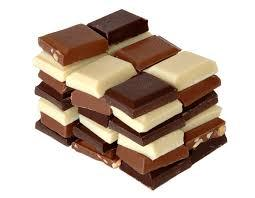Did you find an error or inaccuracy? Feel free to write us. Thank you!Tips to related online calculators
Need help to calculate sum, simplify or multiply fractions? Try our fraction calculator.

## Related math problems and questions:

• Children's home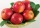The children's home received a gift to Nicholas of 54 oranges, 81 chocolate figurines, and 135 apples. Every child received the same gift and nothing was left. a) How many packages could be prepared? b) what did the children find in the package?
• Chocolate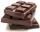How many times do we have to break a chocolate bar composed of 10 × 12 pieces to get the 120 parts?
• 3 children3 children had to divide 4 pounds is candy. How much candy did each child get?
• Sharing chocolateFredericca and her friends share 6 chocolate bars. They *each* get 0.75 of a chocolate bar. How many friends did Fredericca share the chocolate bar with?
• Warehouses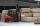To four warehouses is going cement in 25 kg bags. To first one third, to second quarter of the total. The third store got two thirds of the rest, and the last 310 tons came to fourth. How many cement is in all warehouses and how much got every one?
• TrioTrio of workers earn 750 euros. Money divided by the work that each of them made​​. First received twice as the second, the second received three times more than the third. How many got everyone from workers?
• Math classificationIn 3A class are 27 students. One-third got a B in math, and the rest got an A. How many students received a B in math?
• Doses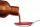A child is to receive a dose of 0.5 teaspoon of cough medicine every 12 hours. if the bottle contains 60 doses, how many days will the medicine last?
• Temperature variationsToday's temperature was 80 degrees, and then the temperature dropped 10 degrees. Then it dropped 15 degrees again, then the next day, the temperature went up 2 degrees. What would the temperature be?
• School trip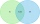On a school trip, 17 of the 28 children bought ice cream or chocolate in a candy store. Twelve children bought chocolate, and nine children bought ice cream. How many children bought ice cream and chocolate? How many children did not buy ice cream? How ma
• Trio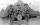56 children lined up in groups of three. How many children did not create a trio?
• DivideDivide substance 110 cm long to two parts so that it first part is 10 cm longer than the second part and one part will be 10 times longer than the second portion. How long the parts will be?
• Apples and pearsMom divided 24 apples and 15 pears to children. Each child received the same number of apples and pears - same number as his siblings. How many apples (j=?) and pears (h=?) received each child?
• Minutes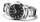Write as fraction in basic form which part of the week is 980 minutes.
• Wood 11Father has 12 1/5 meters long wood. Then I cut the wood into two pieces. One part is 7 3/5 meters long. Calculate the length of the other wood?
• Wire cut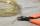A wire of length 9 m was cut into equal lengths using 5 cuts. How long is each piece?
• If-then equationIf 5x - 17 = -x + 7, then x =